## 背景信息

AnalyticDB PostgreSQL支持两种排序方式：

• 组合排序：适用于限定条件是查询的前缀子集或者完全包含排序键，更适合于查询包含首列限定条件的情况。
• 多维排序：给每一个排序键分配相同的权重，更适合于查询条件包含任意限定条件子集的场景。

## 组合排序和多维排序的性能对比

1. 创建测试表并设置表的排序键
``````CREATE TABLE test(id int, num1 int, num2 int, value varchar)
with(APPENDONLY=TRUE, ORIENTATION=column)
DISTRIBUTED BY(id)
SORTKEY(id, num1, num2);

CREATE TABLE test_multi(id int, num1 int, num2 int, value varchar)
with(APPENDONLY=TRUE, ORIENTATION=column)
DISTRIBUTED BY(id)
SORTKEY(id, num1, num2);``````
2. 写入测试数据
``````INSERT INTO test(id, num1, num2, value) select g,
(random()*10000000)::int,
(random()*10000000)::int,
(array['foo', 'bar', 'baz', 'quux', 'boy', 'girl', 'mouse', 'chlid', 'phone'])[floor(random() * 10 +1)]
FROM generate_series(1, 10000000) as g;

INSERT INTO test_multi SELECT * FROM test;

count
----------
10000000
(1 row)

count
----------
10000000
(1 row)``````
3. 对两张表分别进行组合排序和多维排序
``````SORT test;
MULTISORT test_multi;``````
4. 点查询性能对比
• 包含首列排序键限定条件
``````-- Q1 包含首列限定条件
select * from test where id = 100000;
select * from test_multi where id = 100000;``````
• 包含第二列限定条件
``````-- Q2 包含第二列限定条件
select * from test where num1 = 8766963;
select * from test_multi where num1 = 8766963;``````
• 包含二三列限定条件
``````-- Q3 包含二三列限定条件
select * from test where num1 = 100000 and num2=2904114;
select * from test_multi where num1 = 100000 and num2=2904114;``````

性能对比结果：

排序模式 Q1 Q2 Q3
组合排序 0.026s 3.95s 4.21s
多维排序 0.55s 0.42s 0.071s
5. 范围查询性能对比
• 包含首列排序键限定条件
``````-- Q1 包含首列限定条件
select count(*) from test where id>5000 and id < 100000;
select count(*) from test_multi where id>5000 and id < 100000;``````
• 包含第二列限定条件
``````-- Q2 包含第二列限定条件
select count(*) from test where num1 >5000 and num1 <100000;
select count(*) from test_multi where num1 >5000 and num1 <100000;``````
• 包含二三列限定条件
``````-- Q3 包含二三列限定条件
select count(*) from test where num1 >5000 and num1 <100000; and num2 < 100000;
select count(*) from test_multi where num1 >5000 and num1 <100000 and num2 < 100000;``````

性能对比结果：

排序方式 Q1 Q2 Q3
组合排序 0.07s 3.35s 3.64s
多维排序 0.44s 0.28s 0.047s

• 对于Q1场景，由于包含排序键的首列，所以组合排序的效果非常好，而多维排序则会相对性能弱一些。
• 对于Q2场景，由于不包含排序键的首列，组合排序基本上失效了，而多维排序依然能维持比较稳定的性能提升。
• 对于Q3场景，由于不包含排序键的首列，组合排序依然起不到很好的效果，并且由于比较条件的增加，需要额外的比较开销，时间更长，而多维排序表现出更好的性能，这是因为在查询时，限定条件包含的多维排序键越多，性能越好。

## 排序加速计算

• 排序加速计算功能需要数据完全有序，当您写入数据后需要重新执行`SORT <tablename>`对数据进行排序。
• 排序加速计算功能默认开启。

1. 创建测试表far，语句如下：
``````CREATE TABLE far(a int,  b int)
WITH (APPENDONLY=TRUE, COMPRESSTYPE=ZSTD, COMPRESSLEVEL=5)
DISTRIBUTED BY (a)  --分布键
ORDER BY (a);       --排序键``````
2. 写入1000000行数据，语句如下：
``INSERT INTO far VALUES(generate_series(0, 1000000), 1);``
3. 数据导入完成后，对数据进行排序，语句如下：
``SORT far;``

• ORDER BY加速
• 排序加速前（未排序）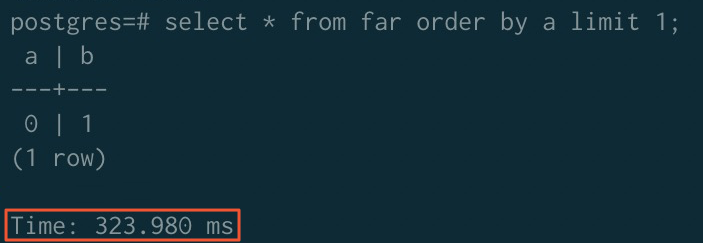• 排序加速后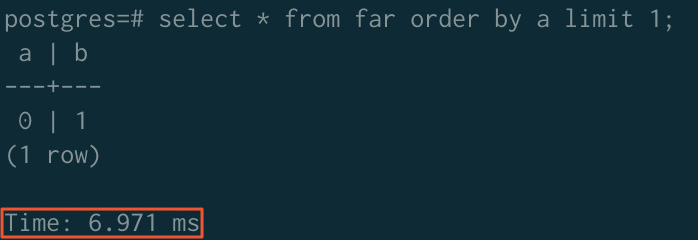• GROUP BY加速
• 排序加速前（未排序）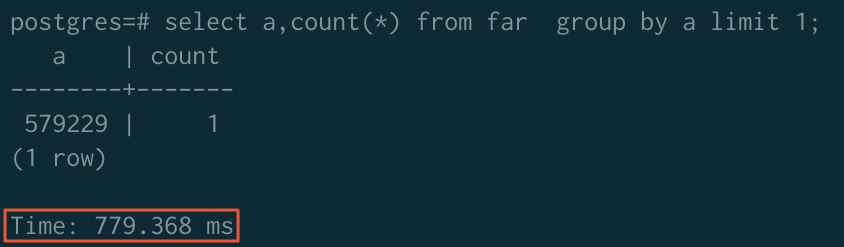• 排序加速后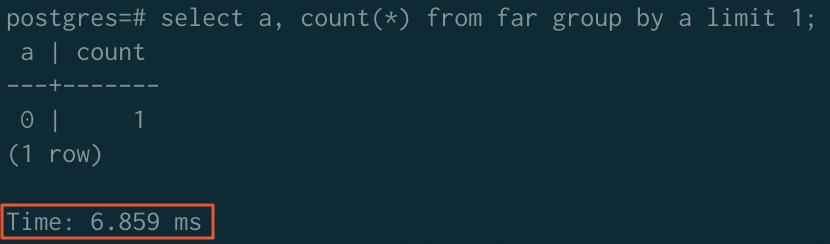• JOIN加速
• 排序加速前（未排序）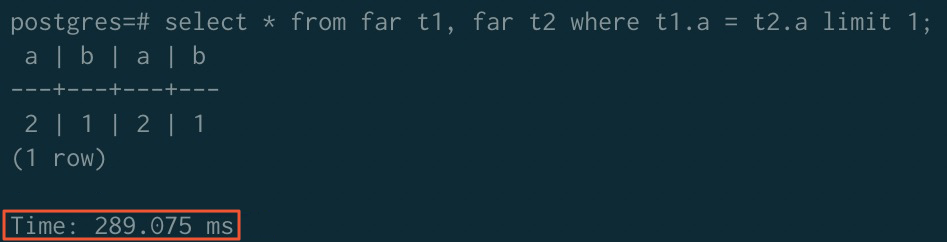• 排序加速后
说明 JOIN排序加速需要关闭ORCA功能，打开mergejoin功能，语句如下：
``````SET enable_mergejoin TO on;
SET optimizer TO off;``````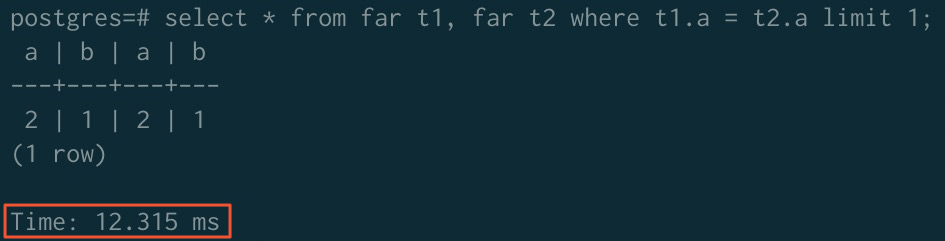- ORDER BY GROUP BY JOIN